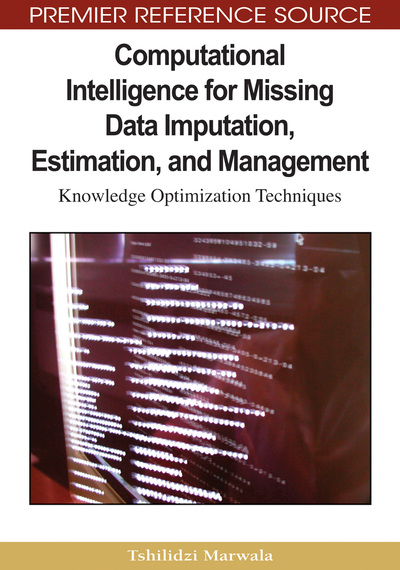# Maximum Expectation Algorithms for Missing Data Estimation

Tshilidzi Marwala
DOI: 10.4018/978-1-60566-336-4.ch004
OnDemand:
(Individual Chapters)
Available
\$37.50
No Current Special Offers

## Abstract

Two sets of hybrid techniques have recently emerged for the imputation of missing data. These are, first, the combination of the Gaussian Mixtures Model and the Expectation Maximization algorithms (the GMM-EM) and second, the combination of Auto-Associative Neural Networks with Evolutionary Optimization (the AANN-EO). In this chapter, the evolutionary optimization method implemented is the particle swarm optimization method (the AANN-PSO). Both the GMM-EM and AANN-EO techniques have been discussed individually and their merits discussed at length in the available literature. This chapter provides a comparison between these techniques, using datasets from an industrial power plant, an industrial winding process and an HIV sero-prevalence survey. The results show that GMMEM method is suitable and performs better in cases where there is little or no interdependency between the input variables, whereas the AANN-PSO combination is suitable when there are inherent nonlinear relationships between some of the given variables.
Chapter Preview
Top

## Introduction

Databases, such as those that store measurement or medical data may become subject to missing values in either the data acquisition or data-storage process. Problems in a sensor, a break in the data transmission line or non-response to questions posed in a questionnaire are prime examples of how data can go missing. The problem of missing data creates a difficulty in the analysis and decision-making processes that depend on the data to be in a complete form and, thereby, they require methods of estimation that are accurate and efficient. Various techniques exist as a solution to this problem, ranging from data deletion to methods employing statistical and artificial intelligence techniques for the imputation of missing variables. However, some statistical methods, like mean substitution have a high likelihood of producing biased estimates (Tresp, Neuneier, & Ahmad, 1995) or make assumptions about the data that may not be true, affecting the quality of decisions based on these data.

The estimation of missing data in real-time applications requires a system that possesses knowledge of characteristics such as correlations between variables, which are inherent in the input space. Computational intelligence techniques and maximum likelihood techniques do possess such characteristics and as a result are important for the imputation of missing data.

This chapter now compares the above two approaches to the problem of missing data estimation. The first technique is based on the combined use of Gaussian Mixture Models with Expectation Maximization, the GMM-EM (Schafer, 1997; Schafer & Olsen, 1998; Schafer & Graham, 2002). The second approach is the use of a system based on the missing data estimation error equation made out of an Auto-Associative Neural Network (Adbella & Marwala, 2005) and solved using Particle Swarm Optimization, the AANN-PSO. A genetic algorithm was used in Chapter II to solve this equation instead of particle swarm optimization. The estimation abilities of both of these techniques will be compared, based on three datasets and conclusions are then drawn.

Stoica, Xu, and Li (2005) observed that the EM algorithm can be rather slow to converge in some problems. Consequently, they introduced a new algorithm called equalization-maximization algorithm for estimating parameters with missing data. They derived an equalization-maximization algorithm in a generalized fashion and implemented this in the case of a Gaussian auto-regressive time series with a varying number of missing observations. They observed that equalization-maximization did out-perform the EM algorithm in terms of computational speed, but did not necessarily do the same in terms of estimating missing data.

Park, Qian, and Jun (2007) proposed an algorithm for estimating parameters that is based on the likelihood function which accounts for the missing information. They assumed a binomial response and normal exploratory model for the missing data and fitted the model by using the Monte Carlo EM algorithm. They derived the Expectation step using the Metropolis-Hastings algorithm to generate a sample for missing data, and for the Maximization step, they maximized the likelihood function using the Newton-Raphson method. They also derived the asymptotic variances and the standard errors of the maximum likelihood estimates by using the observed Fisher information. Furthermore, M’hiri, Cammoun, and Ghorbel (2007) used the EM algorithm in logistic linear models for non-ignorable missing data estimation.

## Complete Chapter List

Search this Book:
Reset